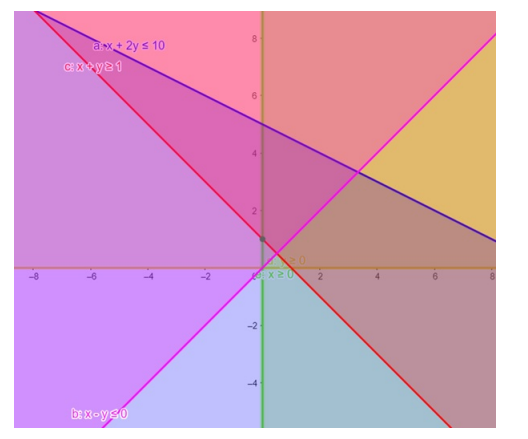# Solve the given inequalities`
Question:

Solve the given inequalities $x+2 y \leq 10, x+y \geq 1, x-y \leq 0, x \geq 0, y \geq 0$ graphically in two - dimensional plane.

Solution:

The graphical representation of $x+2 y \leq 10, x+y \geq 1, y \geq 0$

$x-y \leq 0, x \geq 0$ is given by common region in the figure below.

$x+2 y \leq 10 \ldots \ldots$ (1)

$x+y \geq 1 \ldots \ldots$ (2)

$x \geq 0 \ldots \ldots$ (3)

$y \geq 0$ ……. (4)

$x-y \leq 0 \ldots \ldots(5)$

Inequality (1) represents the region below line $x+2 y=10$ (including the line $x+2 y=10$ ).

Inequality (2) represents the region above line $x+y=1$ (including the line $x+y=1$ ).

Inequality (3) represents the region in front of line $x=0$ (including the line $x=0$ ).

Inequality (4) represents the region above line $y=0$ (including the line $y=0$ ).

Inequality (5) represents the region above line $x-y=0$ (including the line $x-y=0$ ).

Therefore,every point in the common shaded region including the points on the respective lines represents the solution for the given inequalities.

This can be represented as follows,